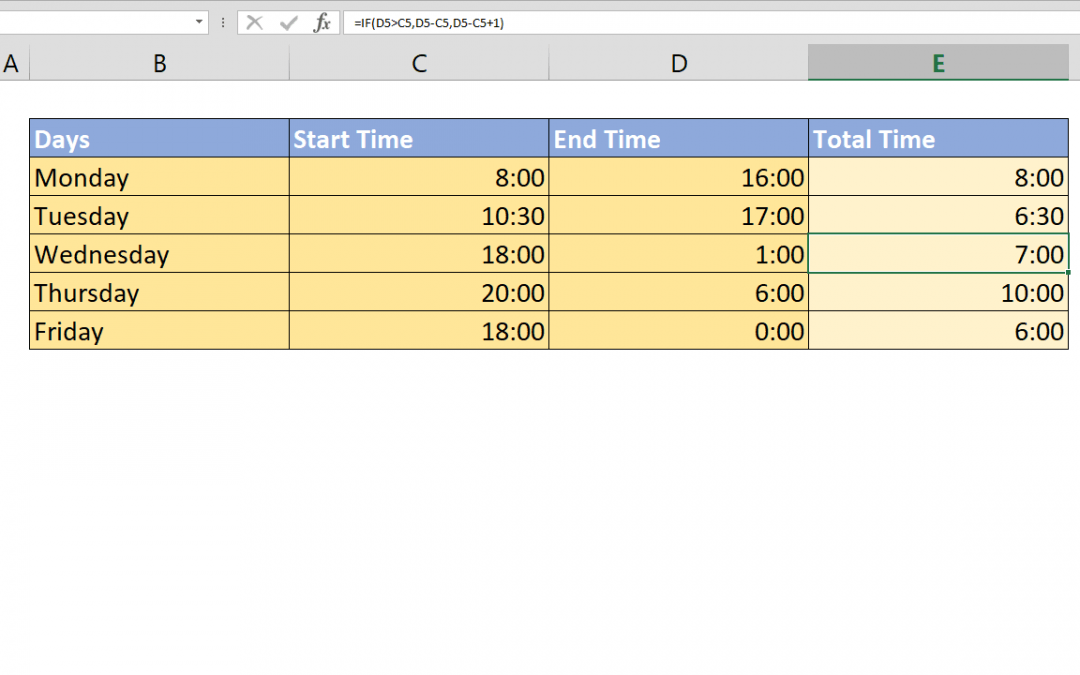Select Page## What week of the year is it and how to get its end date

Although Excel has a function to get week number of a date, WEEKNUM; there isn’t any to make reverse calculation. This method will help you answer the question – what week of the year is it, and give you the end date of each week. Syntax =MIN(DATE(current...## What week of the year is it and how to get its start date

Although Excel has a function to get week number of a date, WEEKNUM; there isn’t any to make reverse calculation. This method will help you answer the question – what week of the year is it, and give you the start date of each week. Syntax...## How to create a time calculator in Excel

When you need to calculate the difference between a time present day and the next day, calculations can get messed up and you will see lots of # characters. This article shows how to create a time calculator in Excel that can calculate shift time after midnight using...## How to calculate average by week number

Calculating averages by week number is a bit different than doing this by month. Instead of finding start and end dates of date range, we focus on the exact week number using the WEEKNUM function. Syntax =AVERAGEIFS( range of values to calculate average, range of week...